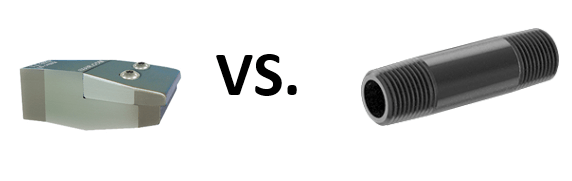EXAIR Air Nozzles – Here’s Their Simple ROIReturn on Investment, or ROI, is the ratio of profit over total investment.  Many people use it to check stocks, financial markets, capital equipment, etc.  It is a quantitative way in determining the validity for an investment or project.   You can use the ROI value to give a measurable rate in looking at your investment.

For a positive ROI value, the project will pay for itself in less than one year.  Any negative values would represent a high-risk investment.  In this blog, I will compare the ROI when replacing a ¼” NPT open pipe with a model 1122 2” Flat Super Air Nozzle.  Let’s start by looking at Equation 1 to calculate the Return on Investment:

Equation 1:  ROI = (Total annual savings – Total Project Cost) / Total Project Cost * 100

The second part of the equation, Total Project Cost, is the cost of the nozzles plus the labor to install them onto the machine.  The model 1122, 2” Flat Super Air Nozzle, has a price of \$70.00 each.  The cost of a ¼” NPT Pipe that is roughly 2” long is around \$1.50 each.  What a difference!  How could EXAIR been in business for over 35 years?  Let’s continue on with the Return on Investment…

The amount of time required to install the nozzles to the end of a pipe is 1/2 hour (generously).  The labor rate that I will use in this example is \$75.00 per hour (you can change this to your current labor rate).  The labor cost to install a nozzle is \$35.00.   The Total Project Cost can be calculated as follows: (\$70 – \$1.50) + \$35.00 = \$103.50.  The next part of the equation, Total annual savings, has more complexity in the calculation, as shown below.

As a reference, EXAIR Super Air Nozzles for compressed air would be considered like LED light bulbs for electricity.  The open pipes and tubes would represent the incandescent light bulbs.  The reason for this parity is because of the amount of energy that the EXAIR Super Air Nozzles can save.  While LED light bulbs are a bit more expensive than the incandescent light bulbs, the Return on Investment has a high percentage, or in other words, a short payback period.  On the other hand, the open pipe is less expensive to purchase, but the overall cost to use in your compressed air system is much much higher.  I will explain why.

To calculate the Total Annual Savings, we need to generate a blow-off scenario (You can use your actual values to calculate the ROI for your project).  In this example, I will compare the ¼” NPT open pipe to the 2” Flat Super Air Nozzle.  (The reason behind this comparison is that the model 1122 can screw directly onto the end of the 1/4” NPT pipe.)   The amount of compressed air used by a 1/4” NPT open pipe is around 140 SCFM (3,962 SLPM) at 80 PSIG (5.5 Bar).  The model 1122 has an air consumption of 21.8 SCFM (622 SLPM) at 80 PSIG (5.5 Bar).  At an electrical rate of \$0.08 per Kilowatt-hour, we see that the cost to make compressed air is \$0.25 per 1000 standard cubic feet, or \$0.25/1000SCF.  (Based on 4 SCFM per horsepower of air compressor).

To calculate an annual savings, let’s use a blow-off operation of 8 hours/day for 250 days a year.   Replacing the ¼” NPT open pipe with a model 1122, it will save you (140 SCFM – 21.8 SCFM) = 118.2 SCFM of compressed air.  To put this into a monetary value, the annual savings will be 118.2 SCFM *\$0.25/1000SCF * 60 Min/hr * 8hr/day * 250 day/yr = \$3,546/year.  Now if you have more than one blow-off spot in your facility like this, imagine the total amount of money that you would save.

With the Total Annual Cost and the Project Cost known, we can insert these values into Equation 1 to calculate the ROI:

ROI = (Total annual savings – Total Project Cost) / Project Cost * 100

ROI = (\$3,546 – \$103.50) / \$103.50 * 100

ROI = 3326%

With a percentage value that high, we are looking at a payback period of only 9 days.  You may look at the initial cost and be discouraged.  But in a little over a week, the model 1122 will have paid for itself.  And after using it for just 1 year, it will save your company \$3,546.00.  Like with any great idea, the LED light bulb clicked on in my mind.  What could be the total savings if you looked at all the blow-off applications in your facility?